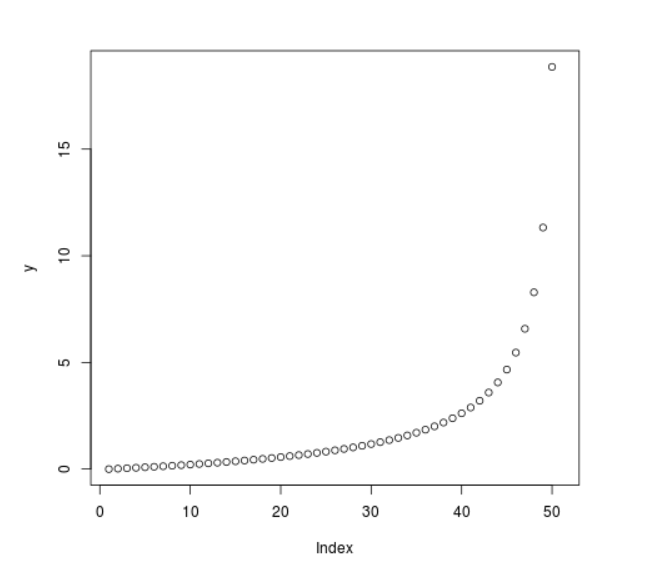Related Articles
Compute the value of Quantile Function over F Distribution in R Programming – qf() Function
• Last Updated : 25 Jun, 2020

`qf()` function in R Language is used to compute the value of quantile function over F distribution for a sequence of numeric values. It also creates a density plot of quantile function over F Distribution.

Syntax: qf(x, df1, df2)

Parameters:
x: Numeric Vector
df: Degree of Freedom

Example 1:

 `# R Program to compute value of``# Quantile Function over F Distribution`` ` `# Creating a sequence of x-values``x <``-` `seq(``0``, ``1``, by ``=` `0.2``)`` ` `# Calling qf() Function``y <``-` `qf(x, df1 ``=` `2``, df2 ``=` `3``)``y`

Output:

``` 0.0000000 0.2405958 0.6085817 1.2630236 2.8860266       Inf
```

Example 2:

 `# R Program to compute the value of``# Quantile Function over F Distribution`` ` `# Creating a sequence of x-values``x <``-` `seq(``0``, ``1``, by ``=` `0.02``)`` ` `# Calling qf() Function``y <``-` `qf(x, df1 ``=` `2``, df2 ``=` `3``)`` ` `# Plot a graph``plot(y)`

Output:My Personal Notes arrow_drop_up